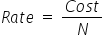Mathematics
Easy

Question

# At the market we get 5 bags of apples for \$20. The cost of 8 bags of apples is ________________.

## 19324213Hint:

## The correct answer is: 32

### Cost of 5 bags of apples is \$20.We have to find the rate. We will find it by taking the ratio of the cost of bags and number of bags. As the rate is constant, we will use this value to find cost of 8 bags of apples.Let the number of bags of apples be denoted as ‘N’.So the equation becomeSo the rate = \$4 per bagLet’s find the cost of 8 bags of applesWe will rearrange this for cost.For this we will multiply both sides by ‘N’.Rate ×N = costCost = Rate × NSubstituting the values we have foundCost = 4 × 8Cost = \$32Therefore, cost of 8 bags of apples is \$32.

We have to be careful about the units. Same quantities should have same units.

### Related Questions to study#### With Turito Foundation.#### Get an Expert Advice From Turito.